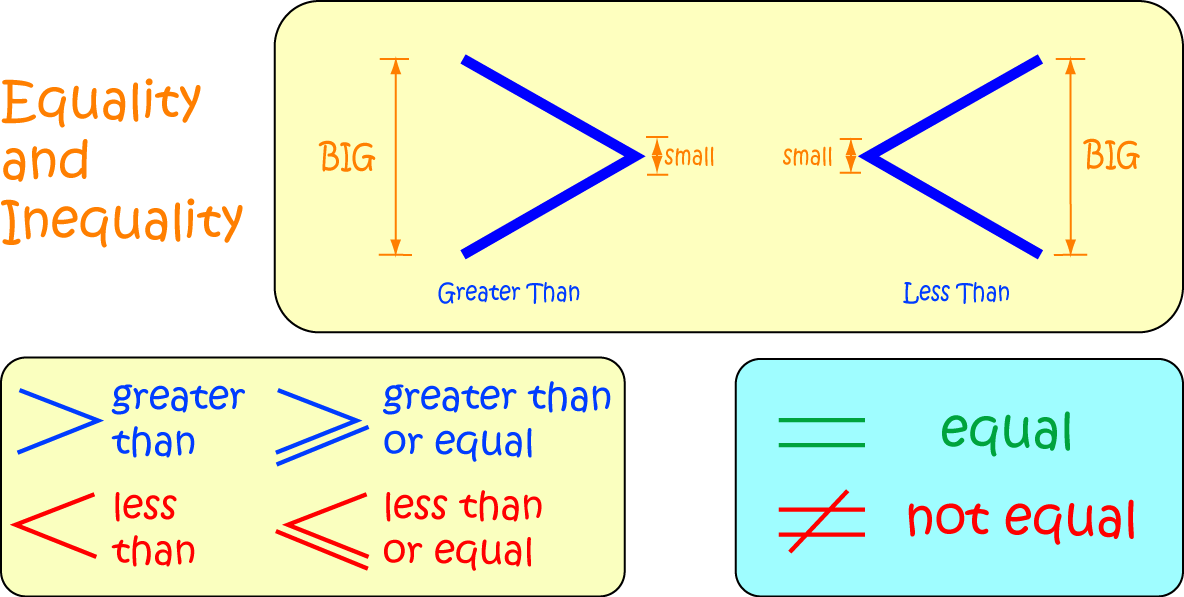# Definition of Not EqualTwo numbers are not equal when they have different values.

We use the symbol $\neq$ to indicate that the numbers or expressions to its left and right are not equal to each other.

For example, $x \neq y$ says that $x$ is not equal to $y$, so $7 \neq 17$ says that $7$ and $17$ have different values.

Another term for "not equal" is "unequal".

### Description

The aim of this dictionary is to provide definitions to common mathematical terms. Students learn a new math skill every week at school, sometimes just before they start a new skill, if they want to look at what a specific term means, this is where this dictionary will become handy and a go-to guide for a student.

### Audience

Year 1 to Year 12 students

### Learning Objectives

Learn common math terms starting with letter N

Author: Subject Coach
You must be logged in as Student to ask a Question.